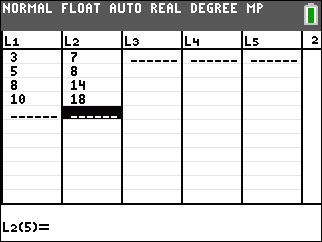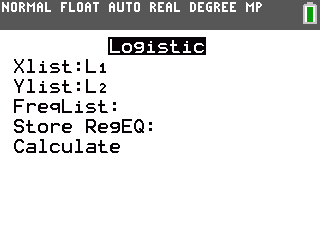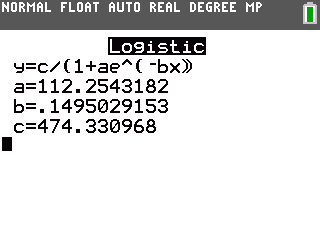# Knowledge Base

## Solution 34492: Calculating Logistic Regressions Using the TI-84 Plus C Silver Edition.

### How do I calculate a logistic regression using the TI-84 Plus C Silver Edition?

The example below will demonstrate how to calculate a logistic regression using the TI-84 Plus C Silver Edition.

Data for this example:

L1: {3,5,8,10}
L2: {7,8,14,18}

1) Press [STAT]  to access the STAT list editor.
2) Enter the data into the L1 and L2 lists, making sure to press [ENTER] after each entry.
3) Press [2nd] [QUIT] to quit and return to the home screen.To calculate the logistic regression:

1) Press [STAT] and scroll right to highlight the CALC menu.
2) Press [ALPHA] [B] to select option B:Logistic.3) Highlight calculate by pressing the down arrow then press [ENTER].

The handheld will display the logistic regression equation in the form y=c/(1+ae^(-bx)). Values will be displayed for the coefficients a, b, and c.Please see the TI-84 Plus C Silver Edition guidebook for additional information.